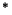Next: SPASS Resolution Proofs Up: Common Syntax of the Previous: Examples

# Proofs

We also define a first, simple proof format. Basically a proof consists of a sequence of ``simple'' steps. The semantics of step is that the introduced formula is a logical consequence of the formulae pointed to by the list of parents.

We already have implemented some scripts that can be used to automatically check resolution proofs. Here, the idea is to be able to check complicated, tedious, long proofs found by some prover automatically by using a different prover.

 proof_list ::= list_of_proof{(proof_type{,assoc_list})}. {step(reference,result,rule_appl,parent_list{,assoc_list}).}end_of_list. reference ::= term | user_reference result ::= term | user_result rule_appl ::= term | user_rule_appl parent_list ::= [parent{,parent}] parent ::= term | user_parent assoc_list ::= [key:value{,key:value}] key ::= term | user_key value ::= term | user_value proof_type ::= identifier | user_proof_typeNext: SPASS Resolution Proofs Up: Common Syntax of the Previous: Examples
Uwe Brahm
1999-09-15